# How to check if an instance of 15 puzzle is solvable?

• Difficulty Level : Hard
• Last Updated : 30 Nov, 2021

Given a 4×4 board with 15 tiles (every tile has one number from 1 to 15) and one empty space. The objective is to place the numbers on tiles in order using the empty space. We can slide four adjacent (left, right, above and below) tiles into the empty space. For example,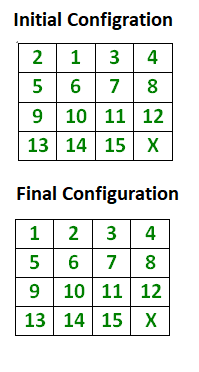Here X marks the spot to where the elements can be shifted and the final configuration always remains the same the puzzle is solvable.
In general, for a given grid of width N, we can find out check if a N*N – 1 puzzle is solvable or not by following below simple rules :

1. If N is odd, then puzzle instance is solvable if number of inversions is even in the input state.
2. If N is even, puzzle instance is solvable if
• the blank is on an even row counting from the bottom (second-last, fourth-last, etc.) and number of inversions is odd.
• the blank is on an odd row counting from the bottom (last, third-last, fifth-last, etc.) and number of inversions is even.
3. For all other cases, the puzzle instance is not solvable.

What is an inversion here?
If we assume the tiles written out in a single row (1D Array) instead of being spread in N-rows (2D Array), a pair of tiles (a, b) form an inversion if a appears before b but a > b.
For above example, consider the tiles written out in a row, like this:
2 1 3 4 5 6 7 8 9 10 11 12 13 14 15 X
The above grid forms only 1 inversion i.e. (2, 1).
Illustration: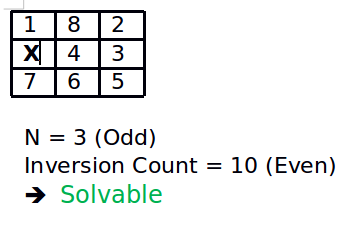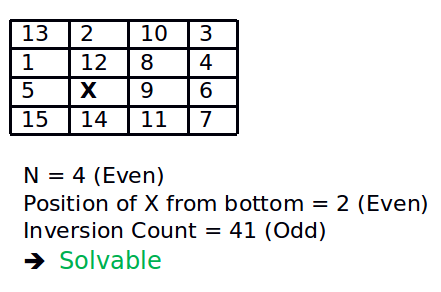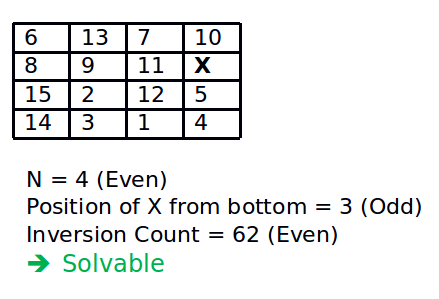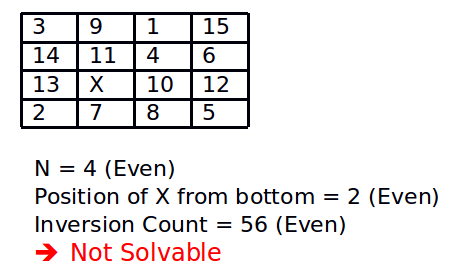Below is a simple C++ program to check whether a given instance of 15 puzzle is solvable or not. The program is generic and can be extended to any grid width.

## C++

 `// C++ program to check if a given instance of N*N-1``// puzzle is solvable or not``#include ``#define N 4``using` `namespace` `std;` `// A utility function to count inversions in given``// array 'arr[]'. Note that this function can be``// optimized to work in O(n Log n) time. The idea``// here is to keep code small and simple.``int` `getInvCount(``int` `arr[])``{``    ``int` `inv_count = 0;``    ``for` `(``int` `i = 0; i < N * N - 1; i++)``    ``{``        ``for` `(``int` `j = i + 1; j < N * N; j++)``        ``{``            ``// count pairs(arr[i], arr[j]) such that``              ``// i < j but arr[i] > arr[j]``            ``if` `(arr[j] && arr[i] && arr[i] > arr[j])``                ``inv_count++;``        ``}``    ``}``    ``return` `inv_count;``}` `// find Position of blank from bottom``int` `findXPosition(``int` `puzzle[N][N])``{``    ``// start from bottom-right corner of matrix``    ``for` `(``int` `i = N - 1; i >= 0; i--)``        ``for` `(``int` `j = N - 1; j >= 0; j--)``            ``if` `(puzzle[i][j] == 0)``                ``return` `N - i;``}` `// This function returns true if given``// instance of N*N - 1 puzzle is solvable``bool` `isSolvable(``int` `puzzle[N][N])``{``    ``// Count inversions in given puzzle``    ``int` `invCount = getInvCount((``int``*)puzzle);` `    ``// If grid is odd, return true if inversion``    ``// count is even.``    ``if` `(N & 1)``        ``return` `!(invCount & 1);` `    ``else`     `// grid is even``    ``{``        ``int` `pos = findXPosition(puzzle);``        ``if` `(pos & 1)``            ``return` `!(invCount & 1);``        ``else``            ``return` `invCount & 1;``    ``}``}` `/* Driver program to test above functions */``int` `main()``{` `    ``int` `puzzle[N][N] =``    ``{``        ``{12, 1, 10, 2},``        ``{7, 11, 4, 14},``        ``{5, 0, 9, 15}, ``// Value 0 is used for empty space``        ``{8, 13, 6, 3},``    ``};``    ``/*``    ``int puzzle[N][N] = {{1, 8, 2},``                    ``{0, 4, 3},``                    ``{7, 6, 5}};` `    ``int puzzle[N][N] = {``                    ``{13, 2, 10, 3},``                    ``{1, 12, 8, 4},``                    ``{5, 0, 9, 6},``                    ``{15, 14, 11, 7},``                ``};` `    ``int puzzle[N][N] = {``                    ``{6, 13, 7, 10},``                    ``{8, 9, 11, 0},``                    ``{15, 2, 12, 5},``                    ``{14, 3, 1, 4},``                ``};`  `    ``int puzzle[N][N] = {``                    ``{3, 9, 1, 15},``                    ``{14, 11, 4, 6},``                    ``{13, 0, 10, 12},``                    ``{2, 7, 8, 5},``                ``};``    ``*/` `    ``isSolvable(puzzle)? cout << ``"Solvable"``:``                        ``cout << ``"Not Solvable"``;``    ``return` `0;``}`

## PHP

 ` arr[j]` `                ``\$inv_count``++;``        ``}``    ``}``    ``return` `\$inv_count``;``}` `// find Position of blank from bottom``function` `findXPosition(``\$puzzle``)``{``    ``global` `\$N``;``    ``// start from bottom-right corner of matrix``    ``for` `(``\$i` `= ``\$N` `- 1; ``\$i` `>= 0; ``\$i``--)``        ``for` `(``\$j` `= ``\$N` `- 1; ``\$j` `>= 0; ``\$j``--)``            ``if` `(``\$puzzle``[``\$i``][``\$j``] == 0)``                ``return` `\$N` `- ``\$i``;``}` `// This function returns true if given``// instance of N*N - 1 puzzle is solvable``function`  `isSolvable( ``\$puzzle``)``{``    ``global` `\$N``;``    ``// Count inversions in given puzzle``    ``\$invCount` `= getInvCount(``\$puzzle``);` `    ``// If grid is odd, return true if inversion``    ``// count is even.``    ``if` `(``\$N` `& 1)``        ``return` `!(``\$invCount` `& 1);` `    ``else`     `// grid is even``    ``{``        ``\$pos` `= findXPosition(``\$puzzle``);``        ``if` `(``\$pos` `& 1)``            ``return` `!(``\$invCount` `& 1);``        ``else``            ``return` `\$invCount` `& 1;``    ``}``}` `/* Driver program to test above functions */`  `    ``\$puzzle` `=``    ``array``(``        ``array``(12, 1, 10, 2),``        ``array``(7, 11, 4, 14),``        ``array``(5, 0, 9, 15), ``// Value 0 is used for empty space``        ``array``(8, 13, 6, 3),``    ``);``    `  `    ``if``(isSolvable(``\$puzzle``)==0)``    ` `            ``echo`  `"Solvable"``;``     ``else``            ``echo`  `"Not Solvable"``;`  `#This code is contributed by aj_36``?>`

## Python3

 `# Python3 program to check if a given instance of N*N-1``# puzzle is solvable or not`  `# A utility function to count inversions in given``# array . Note that this function can be``# optimized to work in O(n Log n) time. The idea``# here is to keep code small and simple.``N``=``4``def` `getInvCount(arr):``    ``arr1``=``[]``    ``for` `y ``in` `arr:``        ``for` `x ``in` `y:``            ``arr1.append(x)``    ``arr``=``arr1``    ``inv_count ``=` `0``    ``for` `i ``in` `range``(N ``*` `N ``-` `1``):``        ``for` `j ``in` `range``(i ``+` `1``,N ``*` `N):``            ``# count pairs(arr[i], arr[j]) such that``            ``# i < j and arr[i] > arr[j]``            ``if` `(arr[j] ``and` `arr[i] ``and` `arr[i] > arr[j]):``                ``inv_count``+``=``1``        ` `    ` `    ``return` `inv_count`  `# find Position of blank from bottom``def` `findXPosition(puzzle):``    ``# start from bottom-right corner of matrix``    ``for` `i ``in` `range``(N ``-` `1``,``-``1``,``-``1``):``        ``for` `j ``in` `range``(N ``-` `1``,``-``1``,``-``1``):``            ``if` `(puzzle[i][j] ``=``=` `0``):``                ``return` `N ``-` `i`  `# This function returns true if given``# instance of N*N - 1 puzzle is solvable``def` `isSolvable(puzzle):``    ``# Count inversions in given puzzle``    ``invCount ``=` `getInvCount(puzzle)` `    ``# If grid is odd, return true if inversion``    ``# count is even.``    ``if` `(N & ``1``):``        ``return` `~(invCount & ``1``)` `    ``else``:    ``# grid is even``        ``pos ``=` `findXPosition(puzzle)``        ``if` `(pos & ``1``):``            ``return` `~(invCount & ``1``)``        ``else``:``            ``return` `invCount & ``1``    `   `# Driver program to test above functions``if` `__name__ ``=``=` `'__main__'``:` `    ``puzzle ``=``[``        ``[``12``, ``1``, ``10``, ``2``,],``        ``[``7``, ``11``, ``4``, ``14``,],``        ``[``5``, ``0``, ``9``, ``15``,], ``# Value 0 is used for empty space``        ``[``8``, ``13``, ``6``, ``3``,],]` `    ``print``(``"Solvable"``) ``if`  `isSolvable(puzzle) ``else` `print``(``"Not Solvable"``)`

Output

`Solvable`

Time Complexity : O(n2)

Space Complexity: O(n)

How does this works?
Fact 1: For a grid of odd width, all legal moves preserve the polarity (even or odd) of the number of inversions.
Proof of Fact 1

• Moving a tile along the row (left or right) doesn’t change the number of inversions, and therefore doesn’t change its polarity.
• Moving a tile along the column (up or down) can change the number of inversions. The tile moves past an even number of other tiles (N – 1). So move either increases/decreases inversion count by 2, or keeps the inversion count same.

Fact 2: For a grid of even width, the following is invariant: (#inversions even) == (blank on odd row from bottom).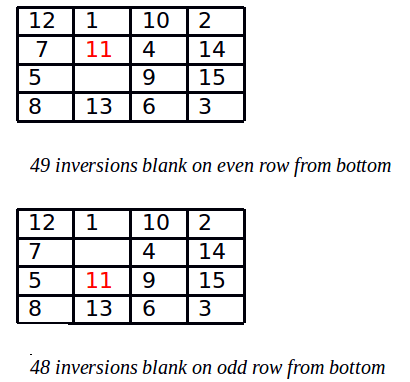Example: Consider the move above. The number of inversions on the left is 49, and the blank is on an even row from the bottom. So the value of the invariant is “false == false”, which is true. The number of inversions on the right is 48, because the 11 has lost two inversions, but the 14 has gained one. The blank is on an odd row from the bottom. So the value of the invariant is “true==true”, which is still true.
Proof of Fact 2

• Moving a tile along the row (left or right) doesn’t change the number of inversions and doesn’t change the row of the blank.
• Moving a tile along the column (up or down) does change the number of inversions. The tile moves past an odd number of other tiles (N – 1). So the number of inversions changes by odd number of times. The row of the blank also changes, from odd to even, or from even to odd. So both halves of the invariant changes. So its value is preserved.

Combining Fact 1 + Fact 2 = Fact 3:

• If the width is odd, then every solvable state has an even number of inversions.
If the width is even, then every solvable state has
• an even number of inversions if the blank is on an odd numbered row counting from the bottom;
• an odd number of inversions if the blank is on an even numbered row counting from the bottom;

Proof of fact 3:

• The initial (solved) state has those properties.
• Those properties are preserved by every legal move.
• Any solvable state can be reached from the initial state by some sequence of legal moves.

Related Article:
How to check if an instance of 8 puzzle is solvable?
Source :
https://www.cs.bham.ac.uk/~mdr/teaching/modules04/java2/TilesSolvability.html